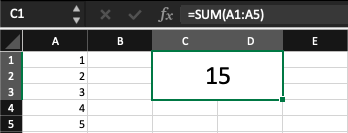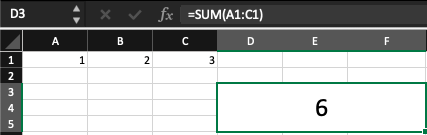# SUM Function

An arithmetic function in excel used for addition.

The Excel SUM function is a quick and easy way to add a range of cells. Simply type "=SUM(" into the cell where you want the result to appear and then select the cells you want to add. Excel will automatically add the cells and show the sum once you hit Enter.One thing to remember is that the SUM function only works on numbers. So, if you have a range of cells that contains both numbers and text, only the numbers will be added.

If you want to add up a range of cells that contains both numbers and text, you can use the SUMIF function. The SUMIF function lets you specify a range of cells to add, ensuring only the cells that meet certain criteria will be added.

You can also use the SUM function to add up multiple ranges of cells. To do this, simply select the first range of cells, type a comma, then select the second range of cells. The function will automatically calculate the sum of both ranges.

## Syntax SUM Function

The Excel SUM function is a quick and easy way to add up a range of cells. Simply type "=SUM(" and then select the cells you want to add.The SUM function in Excel is used to add up a range of cells. The syntax is as follows:

=SUM(beginning_cell:ending_cell)

For example, if you wanted to sum cells A1 through A5, you would use the following syntax:

SUM(A1:A5)You can also use the SUM function on a column or row of cells. For example, to sum cells A1 through C1, you would use the following syntax:

SUM(A1:C1)The SUM function accepts up to 255 arguments. These arguments include numbers, cell references, ranges, arrays, or constants.

The function will return the sum of all the arguments. If any of the arguments are error values, the SUM function will return the #VALUE! Error.

The SUM function is a handy way to quickly add up a range of cells in Excel. Give it a try the next time you need to sum a column or row of cells!### Everything You Need To Master Excel Modeling

To Help You Thrive in the Most Prestigious Jobs on Wall Street.

Researched and authored by Jake Heimowitz | LinkedIn

Reviewed and edited by James Fazeli-Sinaki | LinkedIn